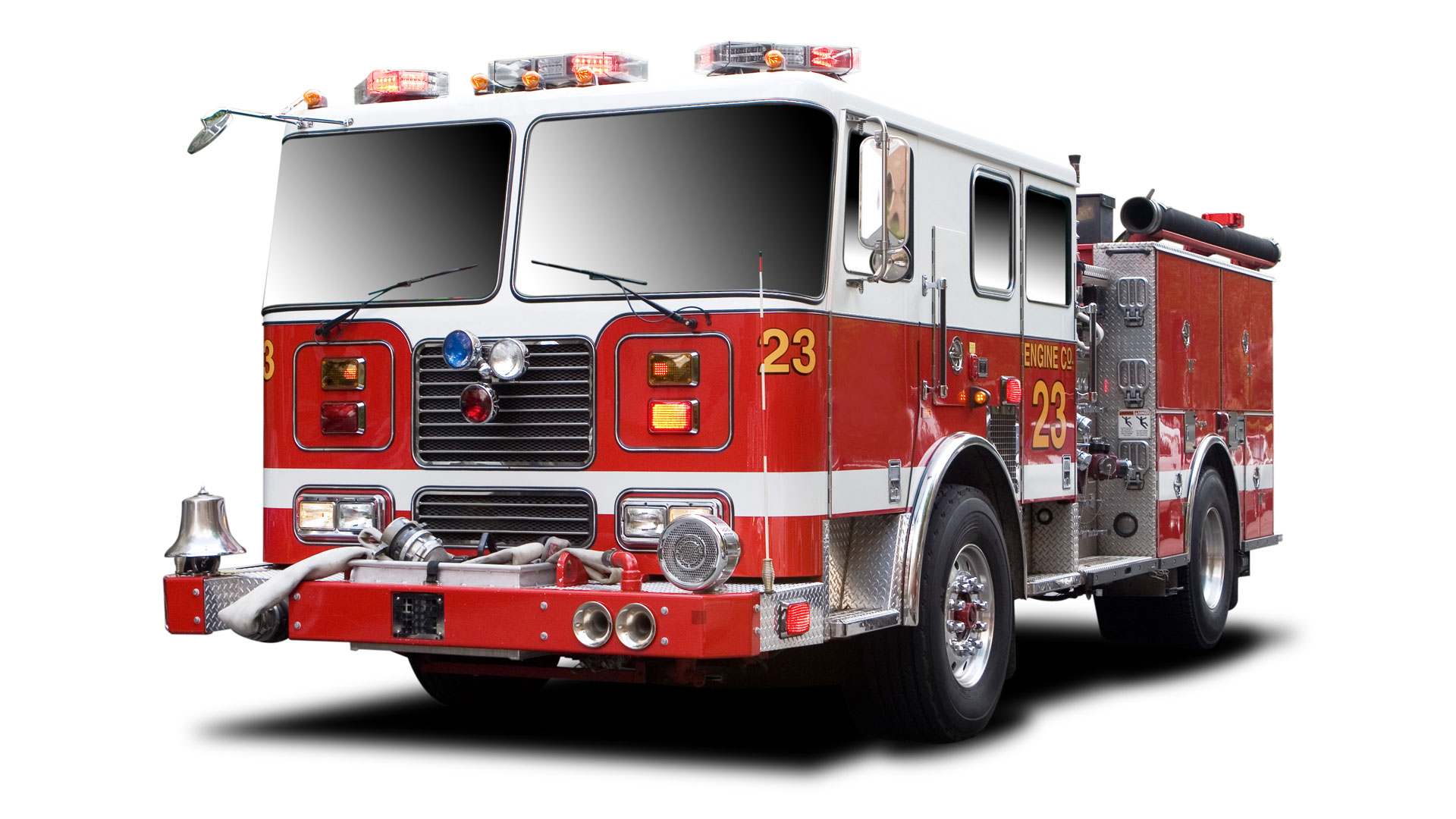# AirPave for Fire Trucks weighing more than 75,000 lbs.

Trey Parker

AirPave Grass Paving for Fire Trucks weighing more than 75,000 lbs.

This would be the responding engine from Corralito’s fire station. Distance between axles:  14’ – 0”Truck #1 Corralito’s 4111 GVWR = 40,540lb

Front Axle = 16,540lbs / 2 wheels = 8,270lbs per tire = 41.35 psi (AirPave 163 times stronger)

Rear Axle = 24,000lbs / 4 wheels = 6,000lbs per tire = 30 psi    (AirPave 224 times stronger)

AirPave Grass Paving

Sand Filled on an appropriate subbase has the compression strength of 6,747 psi = 971,568 psf.

This would be the second engine from Pajaro Valley Fire. Distance between axles:  14’ – 4”Truck #2 Pajaro Valley 4510 GVWR = 38,540lbs

Front Axle = 16,540lbs / 2 wheels = 8,270lbs per tire = 41.35 psi (AirPave 163 times stronger)

Rear Axle = 22,000lbs / 4 wheels = 5,500lbs per tire = 27.5 psi (AirPave 245 times stronger)

AirPave Grass Paving

Sand Filled on an appropriate subbase has the compression strength of 6,747 psi = 971,568 psf.

This would be the “any type” engine from Corralito’s Distance between axles:  15’ – 6”Truck #3 Corralito’s 1174 GVWR = 35,000lbs

Front Axle = 12,000lbs / 2 wheels = 6,000lbs per tire = 30 psi (AirPave 224 times stronger)

Rear Axle = 23,000lbs / 4 wheels = 5,750lbs per tire = 28.75 psi (AirPave 234 times stronger)

AirPave Grass Paving

Sand Filled on an appropriate subbase has the compression strength of 6,747 psi = 971,568 psf.

This would be the Water Tender that would respond Distance to the first axle:  16’ – 1”

Distance to the second axle:  20’ – 9”Truck #4 Water Tender 4551 GVWR = 64,000lbs

Front Axle = 18,000lbs / 2 wheels = 9,000lbs per tire = 45 psi (AirPave 149 times stronger)

Rear Axles = 46,000lbs / 8 wheels = 5,750lbs per tire = 28.75 psi (AirPave 234 times stronger)

AirPave Grass Paving

Sand Filled on an appropriate subbase has the compression strength of 6,747 psi = 971,568 psf.GVWR = 42,000lb

Front Axle = 18,000lbs / 2 wheels = 9,000lbs per tire = 45 psi (AirPave is 6,747 psi)

Rear Axle = 24,000lbs / 4 wheels = 6,000lbs per tire = 30 psi (AirPave is 6,747 psi)

Wheelbase is 180″ between axels on our Engines.

GVWR = 74,980lbs

Front Axle = 21,480lbs / 2 wheels = 10,740lbs per tire = 53.7 psi (AirPave is 6,747 psi)

Inter Axle = 31,000lbs / 4 wheels = 7,750lbs per tire = 38.75 psi (AirPave is 6,747 psi)

Rear Axle = 22,500lbs / 2 wheels = 11,250lbs per tire = 56.25 psi (AirPave is 6,747 psi)

Wheelbase is 176″ between axles on tractor. Total length is 57′ on both trucks.  Let me know if you need anymore information.

Another example…

Let’s say fire truck has a GVWR = 75,000 lb. That weight is distributed amongst the axles and the wheels giving the effective surface pressure.

Using the typical H-20 & H-25 AASHTO formula for Surface Pressure: Axel / number of wheels / 200 square inches of contact per tire (20”x10”) (see below)

Front Axle GVWR = *22,500 lbs / 2 wheels = 11,250 lbs per tire = 56.25psi (AirPave is 120 times stronger)

Rear Axle GVWR = *52,500 lbs / 4 wheels = 13,125 lbs per tire = 65.625psi (AirPave is 103 times stronger)

(*estimated GVWR per axle for example)

We do not have the specs for the 75,000-lb. truck which likely would have 2 rear axles and would drop the psi to 33psi.